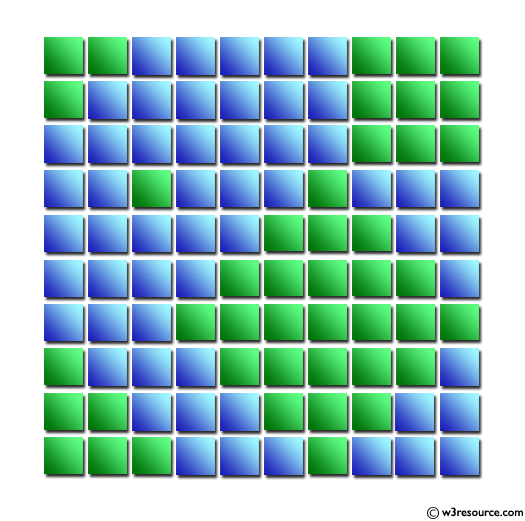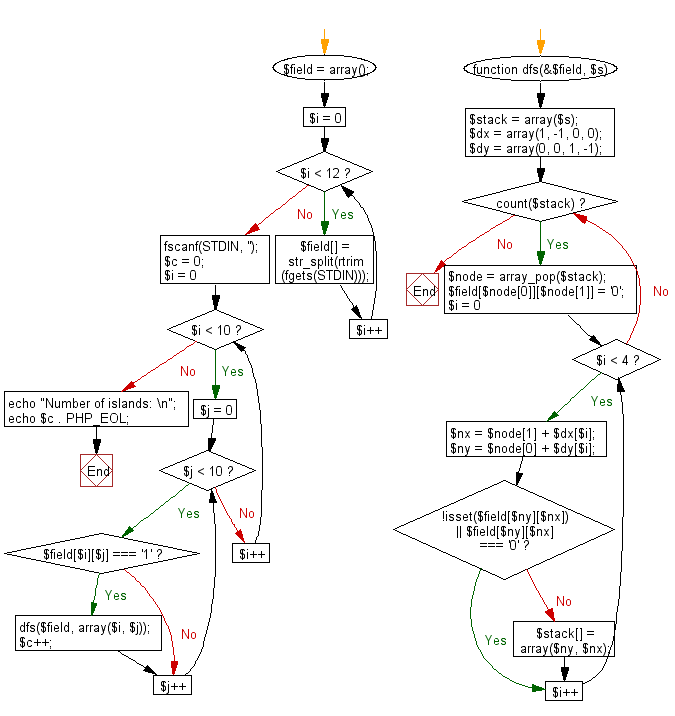﻿ PHP Exercise: Read the mass data and find the number of islands - w3resource

# PHP Exercises: Read the mass data and find the number of islands

## PHP: Exercise-71 with Solution

There are 10 vertical and horizontal squares on a plane. Each square is painted blue and green. Blue represents the sea, and green represents the land. When two green squares are in contact with the top and bottom, or right and left, they are said to be ground. The area created by only one green square is called "island". For example, there are five islands in the figure below.
Write a PHP program to read the mass data and find the number of islands.

Input: A single data set is represented by 10 rows of 10 numbers representing green squares as 1 and blue squares as zeros.

Pictorial Presentation:Sample Solution: -

PHP Code:

``````<?php
{

\$field = array();
for (\$i = 0; \$i < 12; \$i++) {
\$field[] = str_split(rtrim(fgets(STDIN)));
}
fscanf(STDIN, '');

\$c = 0;
for (\$i = 0; \$i < 10; \$i++) {
for (\$j = 0; \$j < 10; \$j++) {
if (\$field[\$i][\$j] === '1') {
dfs(\$field, array(\$i, \$j));
\$c++;
}
}
}

echo "Number of islands: \n";
echo \$c . PHP_EOL;
}

function dfs(&\$field, \$s) {
\$stack = array(\$s);
\$dx = array(1, -1, 0, 0);
\$dy = array(0, 0, 1, -1);
while (count(\$stack)) {
\$node = array_pop(\$stack);
\$field[\$node][\$node] = '0';
for (\$i = 0; \$i < 4; \$i++) {
\$nx = \$node + \$dx[\$i];
\$ny = \$node + \$dy[\$i];
if (!isset(\$field[\$ny][\$nx]) || \$field[\$ny][\$nx] === '0') {
continue;
}
\$stack[] = array(\$ny, \$nx);
}
}
}
?>
```
```

Sample Input:
1100000111
1000000111
0000000111
0010001000
0000011100
0000111110
0001111111
1000111110
1100011100
1110001000

Sample Output:

```Number of islands:
5```

Flowchart:PHP Code Editor:

Have another way to solve this solution? Contribute your code (and comments) through Disqus.

What is the difficulty level of this exercise?

Test your Programming skills with w3resource's quiz.

﻿

## PHP: Tips of the Day

Returns all elements in an array except for the first one

Example:

```<?php
function tips_tail(\$items)
{
return count(\$items) > 1 ? array_slice(\$items, 1) : \$items;
}

print_r(tips_tail([1, 5, 7]));
?>
```

Output:

```Array
(
 => 5
 => 7
)
```